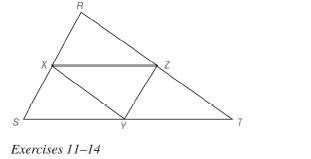Chapter 4.2, Problem 11E### Elementary Geometry for College St...

6th Edition
Daniel C. Alexander + 1 other
ISBN: 9781285195698

#### Solutions

Chapter
Section### Elementary Geometry for College St...

6th Edition
Daniel C. Alexander + 1 other
ISBN: 9781285195698
Textbook Problem
1 views

# In Exercises 11 to 14 , assume that X , Y , and Z are midpoints of the sides of ∆ R S T .If R S = 12 , S T = 14 , and R T = 16 , find: a) X Y b) X Z c) Y ZTo determine

a)

To find:

The value of XY

Explanation

Approach:

The line segment that joins the midpoints of two sides of a triangle is parallel to the third side and has a length equal to one half the length of the third side.

Calculation:

Given,

X, Y, and Z are the midpoints.

RS=12,

ST=14, and

RT=16

Here, X and Y are the midpoints of the sides RS and ST of the triangle RST

To determine

b)

To find:

The value of XZ.

To determine

c)

To find:

The value of YZ.

### Still sussing out bartleby?

Check out a sample textbook solution.

See a sample solution

#### The Solution to Your Study Problems

Bartleby provides explanations to thousands of textbook problems written by our experts, many with advanced degrees!

Get Started

#### Which of the following three graphs is the graph of ? Explain your choice.

Mathematical Applications for the Management, Life, and Social Sciences

#### In Exercises 99-106, factor out the greatest common factor from each expression. 105. 2x5/232x3/2

Applied Calculus for the Managerial, Life, and Social Sciences: A Brief Approach

#### Find numbers a and b such that limx0ax+b2x=1.

Single Variable Calculus: Early Transcendentals

#### Write the following numbers in word form. .00938

Contemporary Mathematics for Business & Consumers

#### Prove the Cauchy-Schwarz inequality |u.v|uv.

Calculus: Early Transcendental Functions (MindTap Course List)

#### Find for y defined implicity by .

Study Guide for Stewart's Multivariable Calculus, 8th